# Probability & Permutation and Combination Quiz for IBPS , SBI , RBI: Quiz – 1

## Probability & Permutation and Combination Quiz for IBPS , SBI , RBI: Quiz – 1

Probability & Permutation and Combination plays a significant role in Quantitative Aptitude Section of banking exams such as IBPS, SBI and RBI PO and Clerk. You will get at least 2-3 questions from Probability & Permutation and Combination in one of IBPS, SBI and RBI PO & clerk exam. So, aspirants should focus on Probability & Permutation and Combination questions in detail. Here, we are providing you with the Probability & Permutation and Combination questions quiz with the detailed solution so that you can easily prepare for Probability & Permutation and Combination questions. We are providing here all-important latest pattern-based questions and Previous Year Questions of Probability & Permutation and Combination of various Government Exam like IBPS, SBI, and RBI PO and Clerk exam. This Probability & Permutation and Combination quiz we are providing is free. Attempt this Probability & Permutation and Combination quiz to practice important questions with answers and solutions. And score better in IBPS, SBI and RBI PO and Clerk exam.

Probability & Permutation and Combination Quiz to improve your Quantitative Aptitude for SBI Po & SBI clerk exam, IBPS PO & IBPS Clerk exam, IBPS RRB PO and assistant exam, LIC AAO, LIC Assistant and other competitive exam.

1. 3 bags, each contains 3 red and 3 white balls. Find the probability of selecting 2 white balls from the same bag?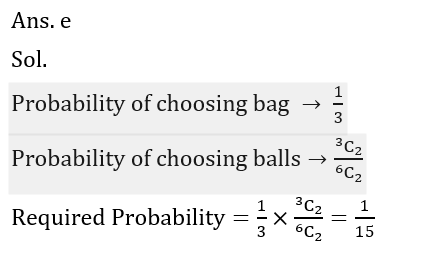2. What is the probability of getting a pair of even cards when two cards draw from a well shuffled pack of card?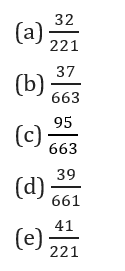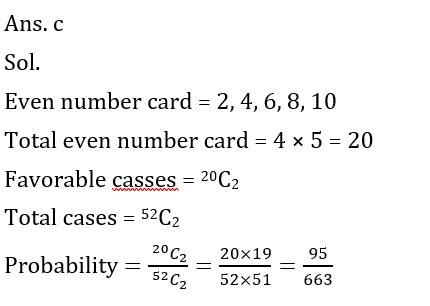3. A five letter word is formed by using first 8 letters of English alphabets. If only one vowel used in the word, then find the total number of ways to form the word without any repetition.

(a) 3800

(b) 1440

(c) 7200

(d) 4800

(e) 36004. In a basket there are 3 papayas and 5 oranges. If one fruit drawn at random from the basket, then find the probability that it is either papaya or orange?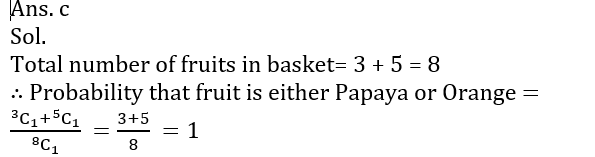5. There are total 36 fruits in a basket in which some are banana and some are Apple. If one fruit is taken out at random and probability of getting an Apple is then find the number of bananas in the basket.

(a) 20

(b) 28

(c) 24

(d) 30

(e) 166. How many 4 digits number can be formed using 2, 3, 5, 7, 6 and 9 if the number should be divisible by ‘4’ and repetition is not allowed?

(a) 120

(b) 96

(c) 160

(d) 64

(e) 2967. In how many different ways can a team of 5 students be formed from 5 girls and 6 boys having at least 1 girl and 2 boys?

(a) 365

(b) 425

(c) 385

(d) 405

(e) 415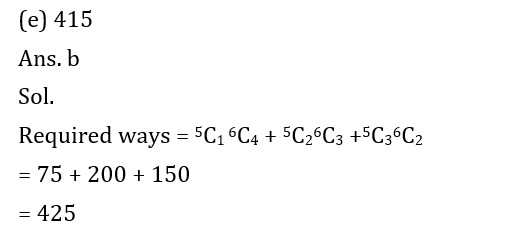8. Find the probability of selecting two face cards or two number cards from a pack of 52 cards?

(a) 61 : 77

(b) 121 : 212

(c) 60 : 121

(d) 116 : 221

(e) 125 : 221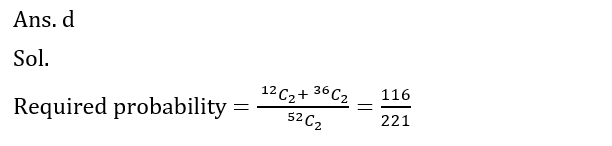9. A bag contain 6 red balls, 3 green balls and 4 blue balls. Find the probability of selecting 3 balls such that 2 balls are of same colour ?10. What are the ways in which a committee of 7 people can be formed from a group of 5 girls and 6 boys such that the committee will always have more number of boys than girls?

(a) 210

(b) 200

(c) 270

(d) 215

(e) 220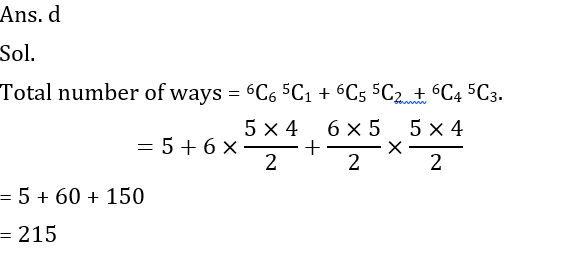#### Attempt Quantitative Aptitude Topic Wise Online Test Series

Recommended PDF’s for :

### Preparation Kit PDF

#### Most important PDF’s for Bank, SSC, Railway and Other Government Exam : Download PDF Now

AATMA-NIRBHAR Series- Static GK/Awareness Practice Ebook PDF Get PDF here
The Banking Awareness 500 MCQs E-book| Bilingual (Hindi + English) Get PDF here
AATMA-NIRBHAR Series- Banking Awareness Practice Ebook PDF Get PDF here
Computer Awareness Capsule 2.O Get PDF here
AATMA-NIRBHAR Series Quantitative Aptitude Topic-Wise PDF 2020 Get PDF here
Memory Based Puzzle E-book | 2016-19 Exams Covered Get PDF here
Caselet Data Interpretation 200 Questions Get PDF here
Puzzle & Seating Arrangement E-Book for BANK PO MAINS (Vol-1) Get PDF here
ARITHMETIC DATA INTERPRETATION 2.O E-book Get PDF here

3